# Gum PolesCCA Treated H4

Diameter of poles, in millimeter – Measured at the top end of each Pole

Click on the desired length below to view price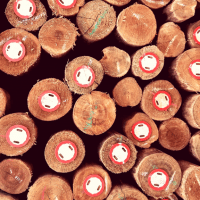Red Tag 50-75mm
50-75mm
• R17.48 Each

• R22.56 Each

• R27.90 Each

• R33.57 Each

• R39.54 Each

• R45.79 Each

• R52.38 Each

• R66.52 Each

• R81.99 Each

• R98.88 Each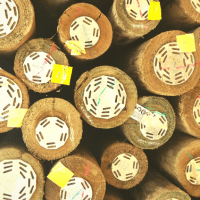Yellow Tag 75-100
75-100mm
• R33.07 Each

• R42.25 Each

• R51.88 Each

• R61.89 Each

• R72.31 Each

• R83.12 Each

• R94.38 Each

• R118.15 Each

• R143.67 Each

• R171.03 Each

• R200.23 Each

• R231.38 Each

• R270.28 Each

• R299.53 Each

non stock length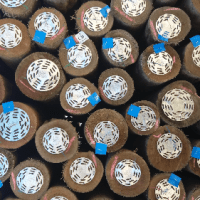Blue Tag 100-125
100-125mm
• R48.88 Each

• R62.17 Each

• R75.95 Each

• R90.18 Each

• R104.88 Each

• R120.07 Each

• R135.71 Each

• R168.45 Each

• R203.17 Each

• R239.95 Each

• R278.78 Each

• R319.75 Each

• R362.84 Each

• R408.18 Each

• R455.80 Each

non stock length

• R505.71 Each

• R557.98 Each

• R650.49 Each

non stock length

• R749.93 Each

non stock length

• R856.44 Each

non stock length

• R970.35 Each

non stock length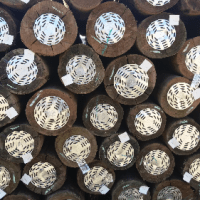White Tag 125-150
125-150mm
• R69.92 Each

• R88.73 Each

• R108.02 Each

• R127.90 Each

• R148.27 Each

• R169.22 Each

• R190.73 Each

• R235.40 Each

• R282.37 Each

• R331.71 Each

• R383.38 Each

• R437.52 Each

• R510.46 Each

• R553.18 Each

non stock length

• R614.86 Each

non stock length

• R679.12 Each

non stock length

• R746.09 Each

non stock length

• R863.64 Each

non stock length

• R988.95 Each

non stock length

• R1122.22 Each

non stock length

• R1263.61 Each

non stock length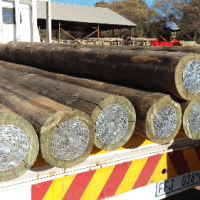### Mostly on order

Orange Tag 150-175
150-175mm
• R96.78 Each

non stock length

• R122.51 Each

non stock length

• R148.86

non stock length

• R175.83 Each

non stock length

• R203.44 Each

non stock length

• R231.71 Each

• R260.61 Each

• R320.37 Each

• R382.78 Each

non stock length

• R447.89 Each

non stock length

• R515.74 Each

non stock length

• R586.41 Each

non stock length

• R659.87 Each

non stock length

• R736.25 Each

non stock length

• R815.57 Each

non stock length

• R897.83 Each

non stock length

• R983.11 Each

non stock length

• R1132.12 Each

non stock length

• R1289.89 Each

non stock length

• R1456.59 Each

non stock length

• R1632.41 Each

non stock length### Order Only

No Tag 175-200 +
175-200mm
• R111.28 EX-VAT

• R206.44 Each

• R250.11 Each

• R294.61 Each

• R339.92 Each

• R386.03 Each

• R433.00 Each

• R529.46 Each

• R629.27 Each

• R732.53 Each

• R839.25 Each

• R949.49 Each

• R1063.31 Each

• R1180.75 Each

• R1301.86 Each

• R1426.66 Each

• P.O.A

• R1777.95 Each

• R2011.43 Each

• P.O.A

• P.O.A

##### All the prices displayed on this page is Including 15% VAT. We do our best to keep our website prices up to date, However prices are subject to change without notice. Please contact us for an official quote and to verify availability.

All poles supplied by Lynnridge Timbers are CCA treated and SABS approved. (CCA) Chromated copper arsenate is a wood preservative that has been used for timber treatment since the mid-1930s. It is a mix of chromium, copper and arsenic.

The chromium acts as a chemical fixing agent, it helps the other chemicals to fix in the timber, binding them through chemical complexes to the wood’s cellulose and lignin. The copper acts primarily to protect the wood against decay, fungi, and bacteria, while the arsenic is the main insecticidal component of CCA, providing protection from wood attacking insects including termites and marine borers.

Each pole supplied by Lynnridge Timbers is CCA treated level H4. In accordance with SANS 457.  Level H4 treated poles are suitable for ground contact and periodic wetting. Often used by the Construction Industry, Equine Industry, Municipalities as for the Homeowner and Gardening enthusiast.

Diameter of poles, in millimeter – Measured at the top end of each Pole

| 50/75 | 75/100 | 100/125 |125/150 | 150/175 |175/200 |

( Different Lengths can be cut upon request )

Suited for in ground contact

Saligna gum

#### Scientific name:

Eucalyptus grandis (Myrtaceae)

#### Alternative common names:

bluegum; rose gum; saligna (English); salignabloekom (Afrikaans)